### Home > A2C > Chapter 13 > Lesson 13.1.3 > Problem13-57

13-57.
1. Explain each transformation below in terms of the parent graph f(θ) = tan θ. Homework Help ✎

1. f(θ) = tan θ + 1

2.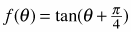3. f(θ) = −tan(θ)

4. f(θ) = 4 tan θ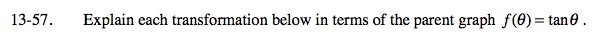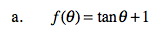Shifted up one unit.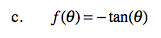Flipped vertically.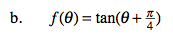$\text{Shifted left }\frac{\pi}{4}\text{ radians.}$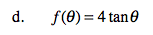Stretched vertically by a factor of 4